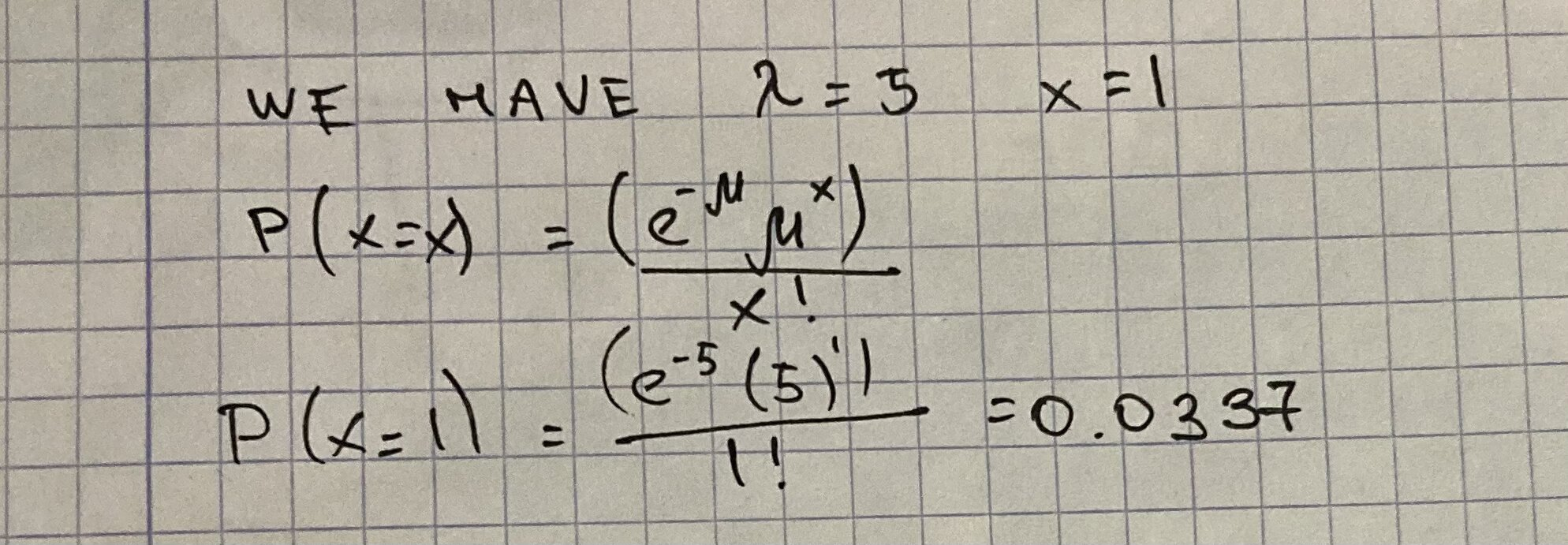Question# Assume a Poisson distribution with lambda = 5.0. What is the probability that X = 1?

Binomial probability
ANSWEREDAssume a Poisson distribution with lambda $$= 5.0$$. What is the probability that $$X = 1$$?